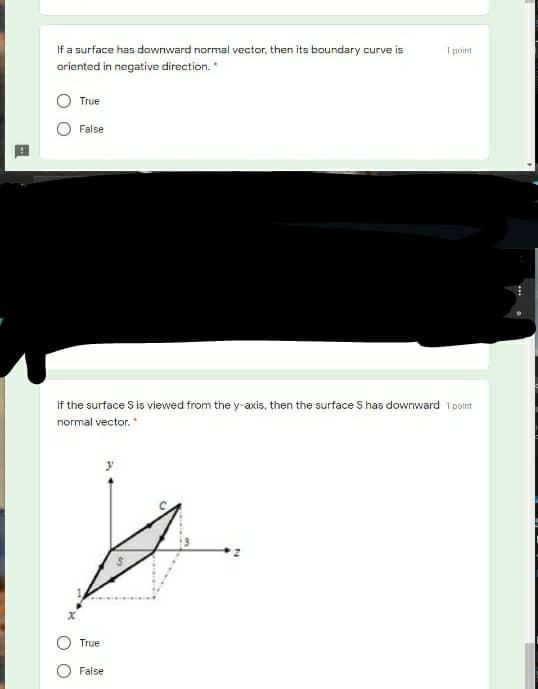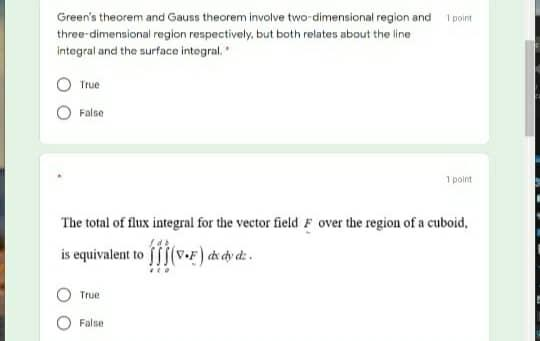### Create an Account

Already have account?

### Forgot Your Password ?

Home / Questions / Toint If a surface has downward normal vector, then its boundary curve is oriented in nega...

# Toint If a surface has downward normal vector, then its boundary curve is oriented in negative direction. True False If the surface S is viewed from the y-axis, then the surface S has downward 1 point

Toint If a surface has downward normal vector, then its boundary curve is oriented in negative direction. True False If the surface S is viewed from the y-axis, then the surface S has downward 1 point normal vector True False
Green's theorem and Gauss theorem involve two-dimensional region and point three-dimensional region respectively, but both relates about the line Integral and the surface integral True False 1 point The total of flux integral for the vector field F over the region of a cuboid, is equivalent to fjs (V-F) diety die True FalseApr 14 2021 View more View Less

#### Answer (Solved)Subscribe To Get Solution